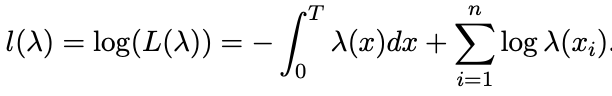# Generative Modelling of non-homogeneous poisson processes

Hello Everyone,

I am an absolute new to probabilistic programming and to the pyro framework. I am trying to model a temporal sequence as a poisson process. My goal is to generate the sequence given fixed model parameters (generative algorithm) and then given the generated sequence I want to infer/fit the model parameters to cross check the inference process.

Generative Model:

I generate a temporal sequence which via a non-homogeneous poisson processes(NHPP). The code for the thinning algorithm to generate the NHPP is given below along with a block on how to run it.

``````def thinning_algo(intensity_function, T, max_intensity = 2):
times = torch.linspace(1e-5, T, 2000)
max_intensity = intensity_function(times).max()
thining_prob = lambda t: intensity_function(t)/max_intensity
t = 0; N = 0; arrival_times = torch.tensor([])
while True:
u1, u2 = pyro.sample("unif", dist.Uniform(0, 1), torch.tensor())
t = t - (u1.log()/max_intensity)
if t < T:
if u2 < thining_prob(t):
arrival_times = torch.cat((arrival_times, torch.tensor([t])))
N += 1
else:
return arrival_times
``````
``````   import numpy
T = 50
#model Parameters to be inferred.
high_rate = 100.0; low_rate = 0.0; phase_length = 25.0 #model parameters which will be inffered.
rate_function = lambda x: low_rate + (high_rate-low_rate)*0.5*(1+numpy.sin(2*numpy.pi*x/phase_length))
arrival_times = thinning_algo(rate_function, T)
``````

The log likelihood of the intensity is given by.

I want MAP estimate of the model parameters given the arrival times and any help would be greatly appreciated. Eventually, I want to have multiple NHPP with different parameters which come from some global prior.